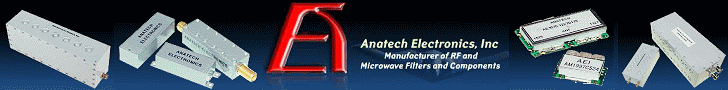About RF CafeCopyright: 1996 - 2024Webmaster:    Kirt Blattenberger,     BSEE - KB3UON RF Cafe began life in 1996 as "RF Tools" in an AOL screen name web space totaling 2 MB. Its primary purpose was to provide me with ready access to commonly needed formulas and reference material while performing my work as an RF system and circuit design engineer. The World Wide Web (Internet) was largely an unknown entity at the time and bandwidth was a scarce commodity. Dial-up modems blazed along at 14.4 kbps while typing up your telephone line, and a nice lady's voice announced "You've Got Mail" when a new message arrived... All trademarks, copyrights, patents, and other rights of ownership to images and text used on the RF Cafe website are hereby acknowledged. My Hobby Website: AirplanesAndRockets.com# Electronics Math QuizNovember 1965 Popular Electronics

 November 1965 Popular ElectronicsTable of Contents Wax nostalgic about and learn from the history of early electronics. See articles from Popular Electronics, published October 1954 - April 1985. All copyrights are hereby acknowledged.

1965 was near the beginning of the transition from vacuum tubes (plasma state) to semiconductors (solid state). If you are not familiar with tube circuits, when deciding what type of mathematical operation is being performed by each circuit, mentally replace the tube with a FET or a BJT. The tube plate becomes the transistor drain or collector, the cathode is the source or emitter, and the control grid is the gate or base, respectively. Don't worry about biasing. Circuits A, D, and I should prove to be the easiest. Circuit F is pretty obvious if you look at the input and output waveforms shown. Anyone familiar with analog power supplies will breeze through circuits B and G (hint: count the number of charge storage components). For the others, ignore that there is a squarewave shown at the input since the function is not dependent on a squarewave to work. For circuit H, mentally reposition the bottom tube above the other and have the B+ line point upward, then figure out what happens as each turns on. It was common to draw B+ lines pointing downward whereas in transistor circuits they normally point upward or sideward. Think of the two tubes in circuit E as parallel resistors changing value (point B+ upward). Circuit C takes a bit of work (hint: the circuit is a detector). Circuit J is the toughest of all, but if you get the other 9 correct, only one possible answer remains from which to choose - that's how I scored 10:10.

## Electronics Math Quiz

By Robert P. Balin

Many basic electronic circuits can and do perform mathematical operations ranging from elementary arithmetic to integral calculus. See if you can identify the electronic circuits (A-J) below which perform the mathematical operations (1-10) at right.2) Count ____________

3) Differentiate ____________

4) Double ____________

5) Divide ____________

6) Integrate ____________

7) Ratio ____________

8) Square ____________

9) Subtract ____________

10) Triple ____________

Quizzes from vintage electronics magazines such as Popular Electronics, Electronics-World, QST, and Radio News were published over the years - some really simple and others not so simple. Robert P. Balin created most of the quizzes for Popular Electronics. This is a listing of all I have posted thus far.

1 - H   Amplifiers which have their load resistors in series produce an output signal proportional to the sum of the in-phase input signals.

2 - F   A step counter produces an escalated output which varies exponentially with the number of pulses it receives. It can be used to count the number of pulses it receives, and as a frequency divider by allowing it to trigger another circuit, say at every 2nd, 3rd ... 7th step, as desired.

3 - I   A differentiator circuit produces an output whose instantaneous values are proportional to the rate of change of the input voltage waveform.

4 - G   A voltage doubler produces a d.c. output which is approximately equal to twice the r.m.s. value of the a.c. input voltage.

5 - D   A voltage divider provides an output which is in the same proportion to the applied voltage as the divider resistance is to the total resistance.

6 - A   An integrating circuit provides an output voltage which is approximately proportional to the time integral and potential of the input voltage.

7 - J   In a ratio detector circuit, the variations of audio frequency output signals have the same ratio as the variations of the applied FM radio frequency signals.

8 - C   A triode square law detector produces an output signal which is proportional to the square of the input signal.

9 - E   A differential amplifier produces an output signal whose amplitude is proportional to the difference between two in-phase input signals.

10 - B   A voltage tripler circuit produces a d.c. output which is approximately equal to three times the r.m.s. value of the a.c. input voltage.

Posted April 25, 2018Please Support RF Cafe by purchasing my  ridiculously low−priced products, all of which I created. These Are Available for Free